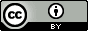## Monday, December 28, 2015

### Standard Gibbs vs Helmholtz Free Energy Change: An Apparent Paradox

Consider the following equilibrium
$$\text{A} + \text{B} \rightleftharpoons \text{AB}$$
and the following three experiments performed at the same temperature.

Experiment 1: To an empty, rigid container with volume $V_1$ is added $p_{\text{A},0}$ of molecule $\text{A}$ and $p_{\text{B},0}$ of molecule $\text{B}$.  The system is allowed to go to equilibrium, $p_{\text{AB},1}$ is measured, and $K_1$ is computed as
$$K_{p,1}=K_1=\frac{p_{\text{AB},1}p^\circ}{p_{\text{A},1}p_{\text{B},1}}$$
where $p_{\text{A},1} = p_{\text{A},0}-p_{\text{AB},1}$

Since the experiment is carried out at constant volume, equilibrium is defined as $dA = 0$ so the standard free energy change computed from $K_1$ should correspond to a standard Helmholtz free energy change, $\Delta A^\circ_1$.

Experiment 2: A balloon is filled with $p_{\text{A},0}$ of molecule $\text{A}$ and $p_{\text{B},0}$ of molecule $\text{B}$. After equilibrium the volume of the balloon is $V_2$ and $p_{\text{AB},2}$ is measured and $K_2$ computed.

Since the experiment is carried out at constant pressure, equilibrium is defined as $dG = 0$ so the standard free energy change computed from $K_2$ should correspond to a standard Gibbs free energy change, $\Delta G^\circ_2$.

Experiment 3: Experiment 1 is repeated using a rigid container with volume $V_2$.

$K$ does not depend on the volume, so $K_3 = K_1$ and $\Delta A^\circ_3=\Delta A^\circ_1$. However, since the temperature and final volume is the same as in Experiment 2, the internal pressure and hence the partial pressures must be the same.  Thus $K_3 = K_2$ and $\Delta A^\circ_3=\Delta G^\circ_2$.  It follows that
$$\Delta A^\circ_1=\Delta G^\circ_2$$

So there is really only one standard free energy change associated with Eq 1, but is it $\Delta G^\circ$ or $\Delta A^\circ$?

The change in standard Helmholz free energy is not the same as the change in standard chemical potential
The equilibrium constant is related to the change in standard chemical potential
$$K = e^{-\Delta \mu^\circ RT}$$
The chemical potential is defined as
$$\mu _X = {\left( {\frac{{\partial G}}{{\partial {n_X}}}} \right)_{p,T,n'}} = {\left( {\frac{{\partial A}}{{\partial {n_X}}}} \right)_{V,T,n'}}$$
For a one-component system, $G = nG_m$, and the chemical potential is simply the molar Gibbs energy of the substance because
$$\mu = {\left( {\frac{{\partial G}}{{\partial {n_X}}}} \right)_{p,T,n'}} = {\left( {\frac{{\partial nG_m^{}}}{{\partial {n_X}}}} \right)_{p,T,n'}} = G_m$$
so we can write
$$K = e^{-\Delta G^\circ RT}$$
where
$$\Delta G^\circ = G^\circ_m(\text{AB)} -G^\circ_m(\text{A)} -G^\circ_m(\text{B)}$$
However, the chemical potential is not equal to the molar Helmholtz free energy
$$A_m = {\left( {\frac{{\partial A}}{{\partial {n_X}}}} \right)_{p,T,n'}} \ne {\left( {\frac{{\partial A}}{{\partial {n_X}}}} \right)_{V,T,n'}}$$
Notice that the middle term is for constant $p$ and the last term is for constant $V$.  So I can't write the equilibrium constant using $\Delta A^\circ$ computed partial molar standard Helmholtz free energies
$$K = {e^{ - \Delta {\mu ^{\circ}}/RT}} \ne {e^{ - \Delta {A^{\circ}}/RT}}$$
Here's why.  The Helmholtz free energy can be written in terms of the partition function $Q=q^N/N!$
$$A = -nRT\ln \left( {\frac{{eq}}{{n{N_A}}}} \right) = -nRT\ln \left( {\frac{{eq_0V}}{{n{N_A}}}} \right)$$
The chemical potential is
$$\mu _X = {\left( {\frac{{\partial A}}{{\partial {n_X}}}} \right)_{V,T,n'}} = - RT\ln \left( {\frac{q}{{n{N_A}}}} \right)$$
To get the partial molar Helmholtz free energy we need to keep $p$ constant, so we substitute $V=nRT/p$ before differentiating, which cancels out the $n$ in the parenthesis
$$A_m = -RT\ln \left( {\frac{{e{q_0}RT}}{{{N_A}p}}} \right) = - RT\ln \left(\frac{eq}{N_A} \right)$$
So from this we can see that
$$\mu_X = A_m + RT$$
In the case of $G$
$$G = A + RT =-nRT\ln \left( {\frac{{q}}{{n{N_A}}}} \right)$$
and we make the substitution $V=nRT/p$ before differentiating to find $\mu$
$$\mu = - RT\ln \left(\frac{q}{N_A} \right) = G_m = A_m + RT$$
So measuring the equilibrium constant for the reaction in Eq 1 gives us
$$\Delta \mu^\circ = \Delta G^\circ = \Delta A^\circ - RT$$